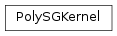# mvpa2.kernels.sg.PolySGKernel¶class `mvpa2.kernels.sg.``PolySGKernel`(**kwargs)

Polynomial kernel: K(a,b) = (a*b.T + c)**degree c is 1 if and only if ‘inhomogenous’ is True

Attributes

 `descr` Description of the object if any

Methods

 `add_conversion`(typename, methodfull, methodraw) Adds methods to the Kernel class for new conversions `as_ls`(kernel) `as_np`() Converts this kernel to a Numpy-based representation `as_raw_ls`(kernel) `as_raw_np`() Directly return this kernel as a numpy array `as_raw_sg`() `as_sg`() `cleanup`() Wipe out internal representation `compute`(ds1[, ds2]) Generic computation of any kernel `computed`(\*args, \*\*kwargs) Compute kernel and return self `reset`()

Initialize instance of PolySGKernel

Parameters: degree : int, optional Polynomial order of the kernel. Constraints: value must be convertible to type ‘int’. [Default: 2] inhomogenous : bool, optional Whether +1 is added within the expression. Constraints: value must be convertible to type bool. [Default: True] use_normalization : bool, optional Optional normalization. Constraints: value must be convertible to type bool. [Default: False] normalizer_cls : sg.Kernel.CKernelNormalizer Class to use as a normalizer for the kernel. Will be instantiated upon compute(). Only supported for shogun >= 0.6.5. By default (if left None) assigns IdentityKernelNormalizer to assure no normalization. normalizer_args : None or list If necessary, provide a list of arguments for the normalizer.

Attributes

 `descr` Description of the object if any

Methods

 `add_conversion`(typename, methodfull, methodraw) Adds methods to the Kernel class for new conversions `as_ls`(kernel) `as_np`() Converts this kernel to a Numpy-based representation `as_raw_ls`(kernel) `as_raw_np`() Directly return this kernel as a numpy array `as_raw_sg`() `as_sg`() `cleanup`() Wipe out internal representation `compute`(ds1[, ds2]) Generic computation of any kernel `computed`(\*args, \*\*kwargs) Compute kernel and return self `reset`()